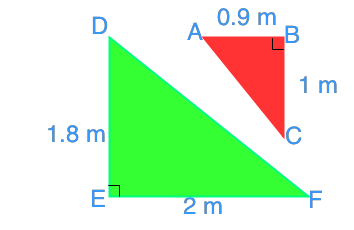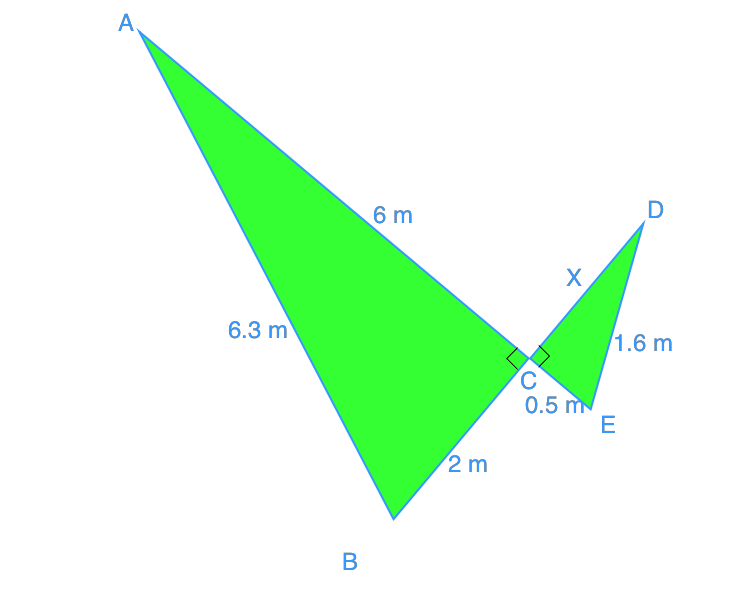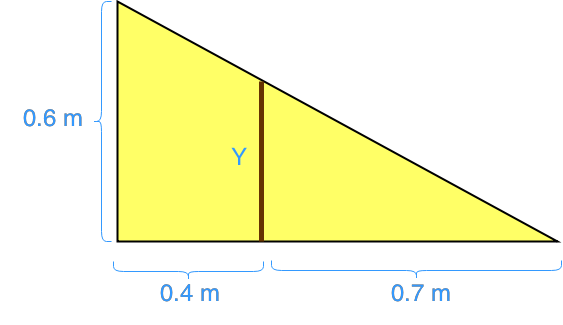# Similar triangles##### Introduction
###### Lessons
1. What are similar triangles?
- common angles
- common ratios (scale factors)
2. What is a scale factor?
##### Examples
###### Lessons
1. Determine if the triangles are similar.1. Which triangles are similar?
Triangle Angles Sides
$\Delta$ABC $\angle$A =90°
$\angle$B =45°
$\angle$C =45°
AB=12.5
BC=17.7
AC=12.5
$\Delta$DEF $\angle$D =90°
$\angle$E =45°
$\angle$F =45°
DE=10
EF=14.2
DF=10
$\Delta$GHI $\angle$G =90°
$\angle$H =60°
$\angle$I =30°
GH=13.8
HI=27.6
GI=23.9
$\Delta$JKL $\angle$J =90°
$\angle$K =45°
$\angle$L =45°
JK=5
KL=7.1
JL=5
1. $\Delta$ABC and $\Delta$DEF are similar triangles. Calculate the missing side length.1. A wood made ramp needs one supporting beam (y) for reinforcement. How long should the supporting beam be?###### Free to Join!
StudyPug is a learning help platform covering math and science from grade 4 all the way to second year university. Our video tutorials, unlimited practice problems, and step-by-step explanations provide you or your child with all the help you need to master concepts. On top of that, it's fun - with achievements, customizable avatars, and awards to keep you motivated.
• #### Easily See Your ProgressWe track the progress you've made on a topic so you know what you've done. From the course view you can easily see what topics have what and the progress you've made on them. Fill the rings to completely master that section or mouse over the icon to see more details.
• #### Make Use of Our Learning Aids###### Practice Accuracy

See how well your practice sessions are going over time.

Stay on track with our daily recommendations.

• #### Earn Achievements as You LearnMake the most of your time as you use StudyPug to help you achieve your goals. Earn fun little badges the more you watch, practice, and use our service.
• #### Create and Customize Your AvatarPlay with our fun little avatar builder to create and customize your own avatar on StudyPug. Choose your face, eye colour, hair colour and style, and background. Unlock more options the more you use StudyPug.
###### Topic Notes
Learn how to determine similar triangles by using side-side-side (SSS) similarity, side-angle-side (SAS) similarity and the properties of similar triangles to solve questions. It's always a good idea to refresh your memory on scale factors and proportions because we will be using them a lot in this lesson.

## Triangle similarity

### define similarity

When you hear that two triangles are similar what does that actually mean? It means that their only difference is their size. Otherwise, their angles are all identical when you match them up! You may see triangles that are flipped, or rotated, but they can still be similar if there's only a difference in their size.

Another thing to note is that with two similar triangles, their corresponding sides have the same ratio. So for example, one triangle may be 1:2 to another triangle, so all their respective sides will be 1:2 to the other triangle.

Let's delve into different ways to prove that two triangles are similar.

## SAS similarity theorem

The SAS similarity theorem stands for side angle side. When you've got two triangles and the ratio of two of their sides are the same, plus one of their angles are equal, you can prove that the two triangles are similar. You've just learned the SAS definition! But there's more...

## SSS similarity theorem

In the SSS similarity theorem, you're looking at proving for the side side side. When you've got two triangles with three sides that have the same ratio, you once again can prove that you've got two similar SSS triangles.

## AA similarity theorem

The AA similarity theorem is named after angle angle. In this case, you can prove that two triangles are similar if two of their corresponding angles are equal. This isn't hard to understand since you know that every triangle's interior angles must equal to 180 degrees. If you've got 2 of the angles figured out, then you know that the last one's value as well. This means essentially that if you know 2 of the angles in two respective triangle are the same, the last angle from the two triangles will be the same as well.

## Example problems

Question 1:

Determine if the triangles are similar:

Solution:

To help you more easily deal with this question, try reorienting the triangles so that they're in a similar orientation. This will help you compare the sides and angles more easily. When you've got this done, let's look at the ratio of the sides of these two triangles.

Ratio of the corresponding sides:

$\frac{DE}{AB}=\frac{1.8}{0.9}=2$

$\frac{EF}{BC}=\frac{2}{1}=2$

Two sides of the triangles have the same ratio. You can also see that there is a 90 angle in both triangles. Therefore, you've proven that they are similar based on the SAS triangle rule.

Question 2:

Which triangles are similar?

A similar triangle must have all the angles equal/congruent. So, the triangle GHI is out of the question. Now, calculate the ratios of the sides. For triangle JKL and DEF, the ratio of their sides is 2. For triangle DEF and ABC, the ratio is 1.25. We can conclude that triangle ABC, DEF , and JKL are similar triangles.

Not convinced about the proofs for similar triangles? See this online interactive diagram that shows you how the angles and the ratio of the sides of two similar triangles change as one triangle gets smaller or bigger.

To help you understand more about triangles, feel free to review the pythagorean theorem and how to use the pythagorean relationship. It's a good stepping stone to help you understand the sides of triangles.

Later on, you'll learn about congruent triangles, how to prove congruence by SSS, as well as SAS and HL, and last but not least, ASA and AAS.